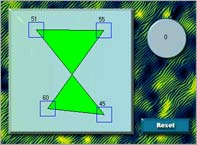Polygon Angle Applet

Description

This java applet was designed to allow students to draw different polygons, and then determine the sum of the angles. First, click a few points on the blue canvas to draw a polygon. After you are done drawing a polygon, click the Drag Angles button, and then click and drag each of the angles over to the angle counter (the blue circle on the right). This angle counter will keep track of the sum of all the angles which have been placed on it.

The purpose of this applet is to show that there is a relationship between the number of vertices and the sum of the angles for convex polygons. See if you can figure it out!Warning: Polygons having lines that cross such as the one pictured here on the left may cause the computer to improperly calculate the size of the angles.

Simulation

Applet Source, Written by Nicholas Exner.

Last Revised: 5/31/00